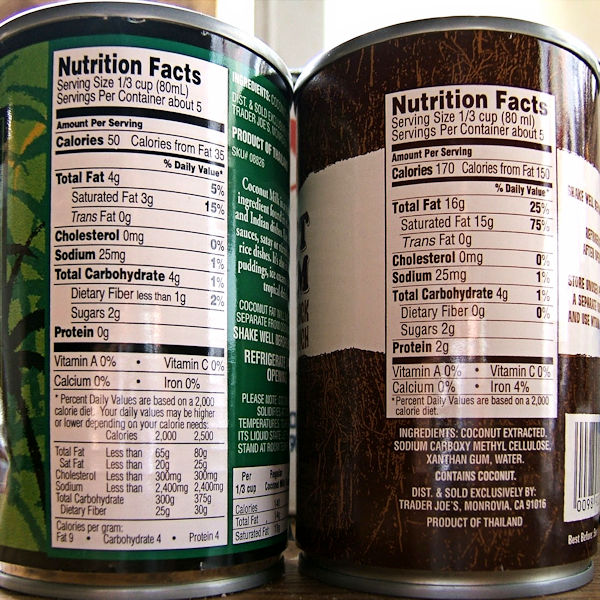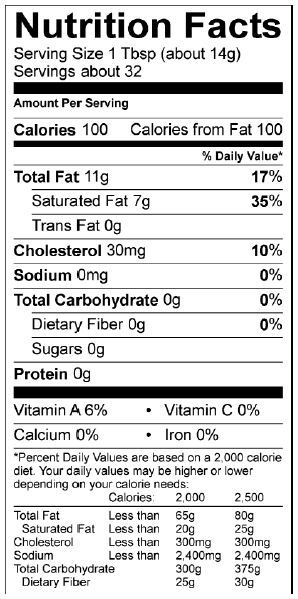# Calculating fat content from nutrition labels

Soap makers sometimes add ingredients such as coconut milk, full-fat milk, cow's cream, and cow's butter to their soap. It is tempting to assume any of these ingredients is all fat or all water.

Sometimes that assumption is fine. For example, it is reasonable to ignore the butterfat in full-fat milk and simply use it as a replacement for water. That assumption will increase the superfat in the soap by only 1% or so.

But that assumption does not always work well for ingredients with a higher fat content, such as butter, cream, or coconut milk. If you ignore the fat in these ingredients, enough "hidden" superfat may be added to the soap to make it overly soft, more prone to rancidity, and less lathersome.

Here is how to read the nutrition information for a product and figure out the amount of fat you will be adding to your soap.

### Example 1. Coconut milk Example 2. Butter

Example 1. Coconut milk

Coconut milk contains two main ingredients as far as soap making is concerned -- water and coconut oil. The water phase may contain dissolved sugars, thickeners, and other additives, but I am going to ignore those ingredients since they are a small portion of the total.

In this example, I am looking at two brands of coconut milk (CM); one is low fat (left) and one is high fat (right). Here are the nutrition facts for both products:Look for two things in this nutrition information -- the total servings in the can and the total fat per serving in grams (g).

To find the total coconut oil in the entire can, multiply the total servings by the amount of fat per serving. In both cases, there are 5 servings per can, but the amount of fat per serving is different:

Total coconut oil per can = Total servings per can X Fat per serving

Total coconut oil per can of Low-fat CM (left) = 5 X 4 grams = 20 grams

Total coconut oil per can of High-fat CM (right) = 5 X 16 grams = 80 grams

Some products are sold by weight, but liquids like coconut milk are sold by volume. So the next thing to do is weigh the total amount of coconut milk in the can. When I did this, the 400 mL of coconut milk weighed about 393 grams.

How much of this coconut milk do you want to use in your soap?

I decided to use a whole can in my soap and I think that is what many soapers do.

So how much is fat and how much is water and other stuff?

We figured out the fat content in the whole can (above) was 20 g for the low fat CM and 80 g for the high fat version. Subtract the fat weight from the total weight to find the amount of water and other non-fat stuff:

Total water per can = Total weight - Total fat

Total water per can of Low-fat CM = 393 - 20 = 373 grams

Total water per can of High-fat CM = 393 - 80 = 313 grams

How much more water do I need in addition to the water supplied by the CM?

In my particular coconut-milk soap recipe, I need a total of 462 grams of water.

Extra water = Total water - Water in the CM

Extra water if using Low-fat CM = 462 - 373 = 89 grams

Extra water if using High-fat CM = 462 - 313 = 149 grams

How much extra coconut oil (CO) do I need in addition to the coconut oil in the milk?

My recipe calls for a total of 250 grams of coconut oil.

Extra CO = Total CO - CO in coconut milk

Extra CO if using Low-fat CM = 250 - 20 = 230 grams

Extra CO if using High-fat CM = 250 - 80 = 170 grams

It is clear the high-fat coconut milk adds a lot of extra coconut oil to the recipe. It does not make sense to ignore this added fat, even though some soapers do.

If I do not include this extra fat as part of the coconut oil in my recipe, how much extra superfat would be added? The total amount of "official" fat in my recipe is 1000 grams.

Extra superfat = Ignored fat / Official fat X 100

Extra superfat if using Low-fat CM = 20 / 1000 X 100 = 2%

Extra superfat if using High-fat CM = 80 / 1000 x 100 = 8%

So if the superfat I want in my soap is 5%, and I ignore the added superfat from the coconut milk, what is the actual total superfat in my soap?

Total superfat = Desired superfat + Ignored superfat

Total superfat if using Low-fat CM = 5% + 2% = 7%

Total superfat if using High-fat CM = 5% + 8% = 13%

Maybe another 2% of "hidden" superfat isn't such a big deal, but what about an extra 8%? Soapers who have tested soap for its ability to lather report a distinct reduction in lather when the superfat is raised 3%. You decide -- does it make sense to ignore this added fat?

Example 2. Cow's butter

Butter contains two main ingredients -- milk fat, also called butterfat, and whey, a watery liquid. As with coconut milk, I will assume the whey is 100% water.

I am going to use the basic calculations shown in Example 1 to calculate the amount of fat and whey in the package of butter and then calculate the fat and whey added to my soap if I use 1 stick (1/4th of a package) of butter to make the soap.How much total milk fat (butterfat) is there in a package of butter?

Total milk fat per package = Total servings X Fat per serving

Total milk fat = 32 X 11 grams = 352 grams

What is the total weight of product in the package?

Butter is sold by weight, because it is a solid, so one way to find the total weight is to look at the front of the package for that information.

In this example, however, I don't have that information because I cannot look at the whole package, but I can still figure this out. The nutrition information says each serving is about 14 grams and there are 32 servings in a package of butter.

Total weight per package = Total servings X Weight per serving

Total weight per package = 32 X 14 = 448 grams

How much of the butter in the package is water (whey)?

Butter contains a lot of fat, but it also contains whey, a watery liquid. You can assume the whey is the same as water.

Total whey per package = Total weight - Total fat

Total whey per package = 448 - 352 = 96 grams

How much fat and whey are in one stick of butter?

If I use 1 stick of butter (one fourth of a package) in my soap, I would divide the total fat in the package by 4 to get the amount of milk fat added to the soap:

Milk fat in 1 stick = 448 / 4 = 112 g

I would also divide the total whey by 4 to get the amount of whey added. I would include this weight as part of the water for my soap:

Whey in 1 stick = 96 / 4 = 24 g